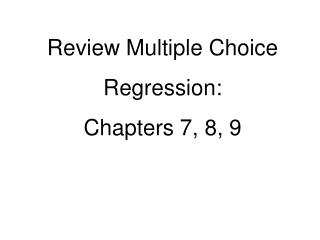DownloadDownload PresentationReview Multiple Choice Regression: Chapters 7, 8, 9

# Review Multiple Choice Regression: Chapters 7, 8, 9

Download Presentation## Review Multiple Choice Regression: Chapters 7, 8, 9

- - - - - - - - - - - - - - - - - - - - - - - - - - - E N D - - - - - - - - - - - - - - - - - - - - - - - - - - -
##### Presentation Transcript

1. Review Multiple Choice Regression: Chapters 7, 8, 9

2. Jay Bennet calculated the regression line for average 1991 SAT scores (total math plus verbal) versus number of dollars spent per student in 1991 for New Jersey school districts and obtained a slope of 0.0227 and a response variable-intercept of 707. • What average SAT result does this regression line predict for students in a district that spends \$10,000 per student? • 467 (B) 480 • (C) 730 • (E) More information is needed to make this calculation. (D) 934

3. Jay Bennet calculated the regression line for average 1991 SAT scores (total math plus verbal) versus number of dollars spent per student in 1991 for New Jersey school districts and obtained a slope of 0.0227 and a response variable-intercept of 707. • 2) According to this analysis, how much should a district spend per student in order for its students to average 1000 on the SAT exam? • \$6,651 • \$16,049 (D) \$44,053 • (E) More information is needed to make this calculation. (B) \$12,907

4. 3) Suppose the regression line for a set of data, passes through point (2, 5). If and are the sample means of the x- and y-values, respectively, then = A) B) C) D) E)

5. 4) Suppose a study finds that the correlation coefficient relating family income to SAT scores is r = + 1. Which of the following are proper conclusions? • I. Poverty causes low SAT scores. • II. Wealth causes high SAT scores. • There is a very strong association between income and SAT scores. • I only (B) II only • (D) I and II • (E) I, II, and III (C) III only

6. 5) Which of the following statements about the correlation coefficient are true? • I. It is not affected by changes in the measurement units of the variables. • II. It is not affected by which variable is called x and which is called y. • It is not affected by extreme values. • (B) I and III • (C) II and III (D) I, II, and III • (E) None of the above gives the complete set of true responses. (A) I and II

7. 6) Which of the following statements about residuals are true? I. The mean of the residuals is always zero. II. The regression line for a residual plot is a horizontal line. III. A definite pattern in the residual plot is an indication that a nonlinear model will show a better fit to the data than the straight regression line. (A) I and II (B) I and III (C) II and III (E) None of the above gives the complete set of true responses. (D) I, II, and III

8. 7) Data are obtained for a group of college freshman examining their SAT scores (math plus verbal) from their senior year of high school and their GPAs during their first year of college. The resulting regression equation is • GPA = 0.00161(SAT) + 1.35 with r = .632 • What percentage of the variation in GPAs can be explained by looking at SAT scores? • (A) 0.161% (B) 16.1% • (D) 63.2% • (E) This value cannot be computed from the information given. (C) 39.9%

9. 8) For a recent English exam, use the Normal model N(73, 9.2) to find the percent of scores over 85. Round to the nearest tenth of a percent. • 88.5% B) 90.3% • C) 11.5% D) 8.1% • E) 9.7%

10. 9) The equation of the least squares regression line for a set of given points is What is the residual for the point (4, 7)? • (B) -2.78 • (C) 4.22 (D) -4.22 • (E) None of these (A) 2.78

11. 10) Tell what the residual plot indicates about the appropriateness of the linear model that was fit to the data. A) Model may not be appropriate. The spread is changing. B) Model is not appropriate. The relationship is nonlinear. C) Model is appropriate.

12. 11) Suppose the regression equation for predicting success on a dexterity task (y) from number of training sessions (x) is and thatWhat percentage of the variation is y is not explained by the regression on x? 34%

13. Chapters 7, 8 and 9: Regression • D 2) B 3) E • 4) C 5) A 6) D • 7) C 8) E 9) A • 10) B 11) 34%